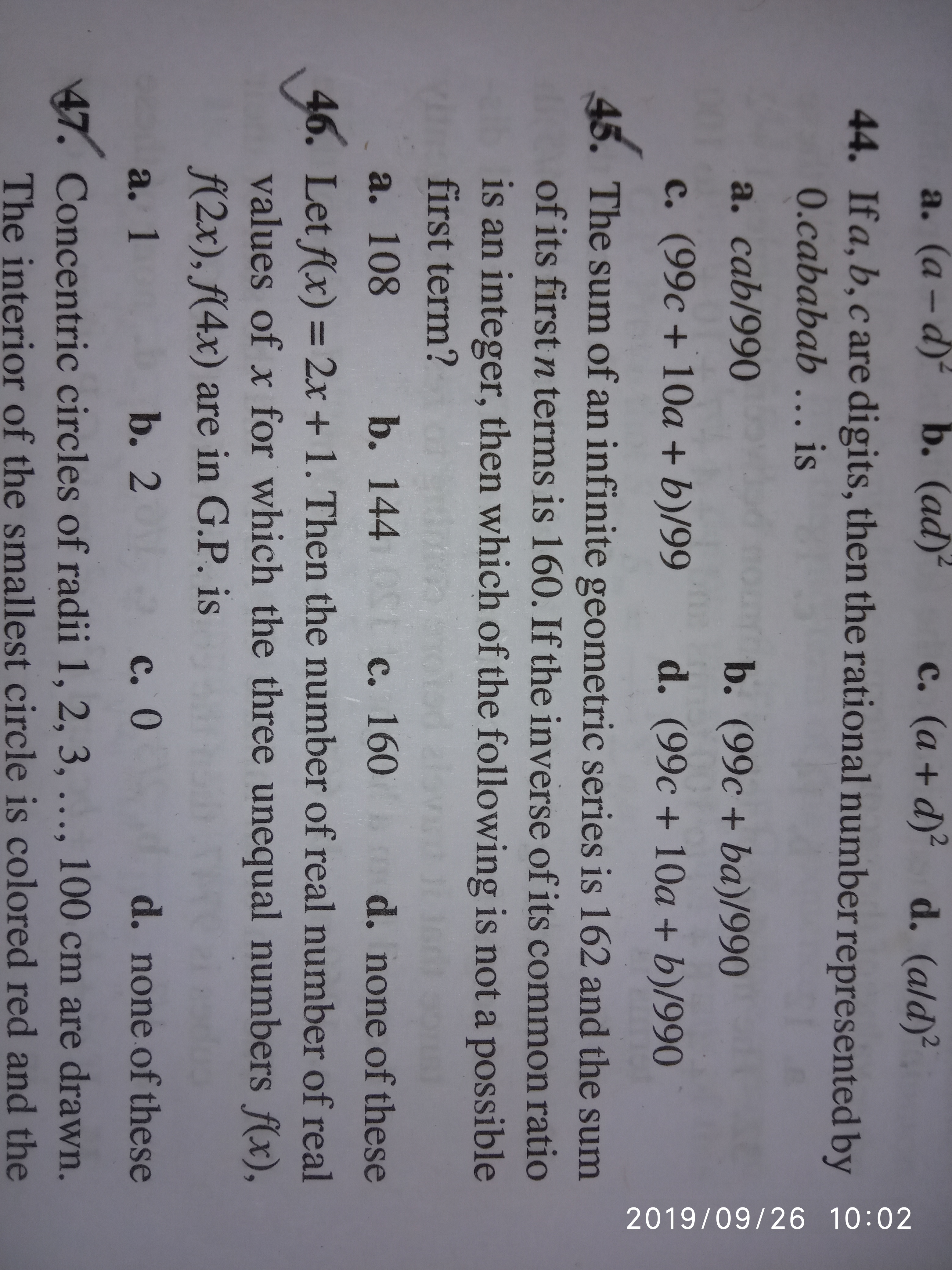# Doubt from Sequence and SeriesQ 45
Just want to confirm the answer, guys just share what are you getting here.

1 Like

It should be D check it.

1 Like

Yeah, getting same but answer mentions option c

Option C will be the answer

Sir, but we can get 160 as first term when r = 1/81?

Why??

2 Likes

Why n=1 is not possible? As there is nothing restriction in question for values of n

if take n=1 then sequence will be like this
G.P= a
How can we say this is G.P

@Shaquib Sir, I don't get you. Can you describe more why it will not an G. P.

If we take n=1 first term of will be 160 and other terms of GP will still exists as r=1/81. I am not getting what you want to say @Shaquib Sir please elaborate

Can you tell How we can declare any constant number a G.P or A.P.

Can you tell what is meaning of sum of first term.

A constant series can be said to be Ap/GP, but here, sum of infinite terms of the gp 160, 160, 160.... is not 162. So, answer is C

Here Series is not repeating it coming as a constant term.

@Hari_Shankar Sir, what are your views here

1 Like

@Tanmay_1 @Shaquib Sir Can value of n change the nature of series from infinite series to non existing series. In this question n is used just to find sum of specific portion of series and not to redefine no of terms in series . Correct me if I am wrong anywhere

@Shreyas_2021 you are too much wrong here
First term is 160 and after that 160/81 and so on
Thanks @Tanmay_1 Sir and @Shaquib sir got it.

1 Like

In this particular case the values of n is changing the series from infinity to non- existing.